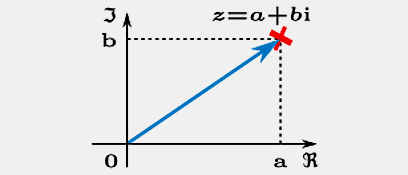complex numbers multiplication
 Question: A сomplex number is represented by z=a+bi , a and b are real numbers and i is the imaginary unit ,satisfying the equation i2 = −1. For more information about complex numers link to: http://blossoms.mit.edu/videos/lessons/complex_numbers_part_imaginary_really_simpleThe polar form of complex numbers: An alternative way of defining a point Z in the complex plane, other than using the x- and y-coordinates, is to use the distance r of the point from O, the point whose coordinates are (0, 0) (the origin ), together with the angle subtended between the positive real axis and the line segment OZ in a counterclockwise direction. This idea leads to the polar form of complex numbers .The pair ( r ,α)  is the polar represntation of the complex number  Another representation of complex number is given by    Watch the video attached and answer the question :In the diagram attached two composite numbers z1,z2 are given : Given that r1>1, r2>1 , Ø1=35° and Ø2=130° . After multipling the given composite numbers the result is z=z1*z2. Of the four options shown , which point represent z ?
3 People tried to answer this question

 The point specified in the letter - ד
 The point specified in the letter - ג
 The point specified in the letter - ב
 The point specified in the letter- א

Correct anwer . good job!!!!

Since each of the multiples is greater than one,  the absolute value of the result is greater than the absolute value of each multiplier.

As we have learned, the angle of the product result is equal to the sum of the multiplier angles.  It follows that Ɵ=Ɵ1+Ɵ2=35+130=165º.

.Watch the movie and repeat the material

You can see that this answer is incorrect : Because each of the multiples is greater than one, their product value is greater than any of them. In the selected point, its distance is too short . In addition, we have learned that the product's angle equals the sum of the ultiples angles. And if you calculate the amount of the given angles you will see that the

location is not suitable.

.Hint: Calculate the sum of the angles and find the appropriate quadrant

Watch the movie and repeat the material .

You can see that this answer is incorrect : Because each of the multiples is greater than one, their product value is greater than any of them. In the selected point, its distance is too short . In addition, we have learned that the product angle equals the sum of the multiples angles. And if you calculate the amount of the given angles you will see that the location is not suitable.

Hint:Calculate the sum of the angles and find the appropriate quadrant

.Watch the movie and repeat the material

We have learned that the product angle equals the sum of the multiples angles. If you calculate the amount of the given angles ,Ɵ1 and Ɵ2, you will see that the chosen location is not suitable.

 1.mualem ola (25.06.2017 am 12.44.27) Peer Assessment: The question relates to the issue of multiplication of complex numbers which is a subject to which many people have not been exposed to before. The introduction of the question (mathematical background), and the mathematical explanation mentioned at the beginning of the video (presentation) enables the students to understand what a complex number is, and at the end of the video the algorithm for multiplication and division in polar representation is mentioned. The question included in most of the criteria for a multi-choice question: background, video, feedback for every disturbance, and the question ends with a question mark. The video: At the beginning of the video it was clarified that the subject is the addition, subtraction, multiplication and division of complex numbers, but throughout the video addition and subtraction were not mentioned. In my opinion, the subject of the video is: complex numbers – definitions, representations, multiplication and division of complex numbers. In addition, between the timeline of 2:02 and 2:25, the teacher stopped talking and explaining, and it was not clear what the purpose of the two sets of axis and representations whose title were: complex numbers in a complex plane, complex numbers, polar representation. It was not clear for me why all the material was written in English and the video was in Hebrew. In my opinion, due to the fact that the site been visited from people all over the world and because the introduction and the question were in English, the video should have been in English. `Responses to the wrong answers with the hints: The responses to the distractors` that are given after the student answers incorrectly encourages the student to continue trying to solve the problem again, with leads to the solution.  It should be mentioned that throughout the question and at all stages, the language must be written in uniform (in this case English) and must be written from left to right.